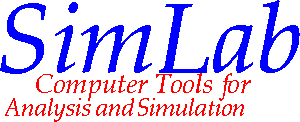# Project Overview

The goal of the Cornell Modeling and Simulation project is to facilitate the construction, modification and evaluation of simulations and other engineering analyses by scientists and engineers. We are creating an environment that permits these processes to be described at an appropriate and natural semantic level. The tools we are developing allow the user to describe the computations using familiar concepts from mathematics and physics, instead of traditional programming languages such as Fortran or C. In addition, we are developing tools that convert these descriptions into efficient codes for sequential and parallel machines.

The approach we have been taking is to integrate the tools and technologies of geometric modeling, symbolic mathematics, numerical analysis, compilation/code generation, and formal methods to create a new methodology and environment for engineering analysis and simulation. These technologies have all been used before to attack engineering analysis problems, but used in isolation. They are far more potent when used in concert within a single integrated environment. Three major components of this approach are discussed below.

• Automate techniques for generating the equations that govern the behavior of physical systems. This includes physical element and variational techniques.

• Develop a language for describing engineering analysis problems based on the natural mathematical and physical concepts of the problem, e.g. differential equations, minimization principles and geometric and topological objects.

• Develop transformation techniques that convert this language into efficient executable code on a variety of different architectures, both sequential and parallel. These re-usable transformations capture mathematical analysis techniques and make them applicable to a wider range of code generation tasks than other approaches. Of particular interest are techniques for meshing geometric objects, discretizing ordinary and partial differential equations and code optimization.